# 6.10 Fields

In modeling applications, particularly those employing FEM methods, situations often arise where it is necessary to describe numeric quantities that vary over some spatial domain. For example, the stiffness parameters of an FEM material may vary at different points within the volumetric mesh. When modeling muscles, the activation direction vector will also typically vary over the mesh.

ArtiSynth provides field components which can be used to represent such spatially varying quantities, together with mechanisms to attach these to certain properties within various materials. Field components can implement either scalar or vector fields. Scalar field components implement the interface ScalarFieldComponent and supply the method

double getValue (Point3d p)

while vector fields implement VectorFieldComponent and supply the method

T getValue (Point3d p)

where T parameterizes a class implementing maspack.matrix.VectorObject. In both cases, the idea is to provide values at arbitrary points over some spatial domain.

For vector fields, the VectorObject represented by T can generally be any fixed-size vector or matrix located in the package maspack.matrix, such as Vector2d, Vector3d, or Matrix3d. T can also be one of the variable-sized objects VectorNd or MatrixNd, although this requires using special wrapper classes and the sizing must remain constant within the field (Section 6.10.4).

Both ScalarFieldComponent and VectorFieldComponent are subclassed from FieldComponent. The reason for separating scalar and vector fields is simply efficiency: having scalar fields work with the primitive type double, instead of the object Double, requires considerably less storage and somewhat less computational effort.

A field is typically implemented by specifying a finite set of values at discrete locations on an underlying spatial grid and then using an interpolation method to determine values at arbitrary points. The field components themselves are defined within the package artisynth.core.fields.

At present, three types of fields are implemented:

Grid fields

The most basic type of field, in which the values are specified at the vertices of a regular Cartesian grid and then interpolated between vertices. As discussed further in Section 6.10.1, there are two main types grid field component: ScalarGridField and VectorGridField<T>.

FEM fields

Fields for which values are specified at the features of an FEM mesh (e.g., nodes, elements or element integration points). As discussed further in Section 6.10.2, there are six primary types of FEM field component: ScalarNodalField and VectorNodalField<T> (nodes), ScalarElementField and VectorElementField<T> (elements), and ScalarSubElemField and VectorSubElemField<T> (integation points).

Mesh fields

Fields in which values are specified for either the vertices or faces of a triangular mesh. As discussed further in Section 6.10.3, there are four primary types of mesh field component: ScalarVertexField and VectorVertexField<T> (vertices), and ScalarFaceField and VectorFaceField<T> (faces).

Within an application model, field deployment typically involves the following steps:

1. 1.

Create the field component and add it to the model. Grid and mesh fields are usually added to the fields component list of a MechModel, while FEM fields are added to the fields list of the FemModel3d for which the field is defined.

2. 2.

Specify field values for the appropriate features (e.g., grid vertices, FEM nodes, mesh faces).

3. 3.

If necessary, bind any needed material properties to the field, as discussed further in Section 6.10.5.

As a simple example of the first two steps, the following code fragment constructs a scalar nodal field associated with an FEM model named fem:

FemModel3d fem;
// ... build the fem ...
ScalarNodalField field = new ScalarNodalField ("stiffness", fem, 0);
for (FemNode3d n : fem.getNodes()) {
double value = ... // compute value for the node
field.setValue (n, value);
}

More details on specific field components are given in the sections below.

## 6.10.1 Grid fields

A grid field specifies scalar or vector values at the vertices of a regular Cartesian grid and interpolates values between vertices. ArtiSynth currently provides two grid field components in artisynth.core.fields:

ScalarGridField
VectorGridField<T>

where T is any class implementing maspack.matrix.VectorObject. For both grid types, the value returned by getValue(p) is determined by finding the grid cell containing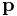and then using trilinear interpolation of the surrounding nodal values. Iflies outside the grid volume, then getValue(p) either returns the value at the nearest grid point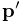(if the component property clipToGrid is set to true), or else returns a special value indicating thatis outside the grid. This special value is ScalarGridField.OUTSIDE_GRID for scalar fields or null for vector fields.

Grid field components are implemented as wrappers around the more basic objects ScalarGrid and VectorGrid<T> defined in maspack.geometry. Applications first create one of these primary grid objects and then use it to create the field component. Instances of ScalarGrid and VectorGrid<T> can be created with constructors such as

ScalarGrid (Vector3d widths, Vector3i res)
ScalarGrid (Vector3d widths, Vector3i res, RigidTransform3d TCL)
VectorGrid (Class<T> type, Vector3d widths, Vector3i res)
VectorGrid (Class<T> type, Vector3d widths, Vector3i res, RigidTransform3d TCL)

where widths gives the grid widths along each of the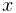,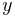and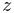axes, res gives the number of cells along each axis, and for vector grids type is the class type of the maspack.matrix.VectorObject object parameterized by T. TCL is an optional argument which, if not null, describes the position and orientation of the grid center with respect to local coordinates; otherwise, in local coordinates the grid is centered at the origin and aligned with the,andaxes.

For the vector grid constructors above, T should be a fixed-size vector or matrix, such as Vector3d or Matrix3d. The variable sized objects VectorNd and MatrixNd can also be used with the aid of special wrapper classes, as described in Section 6.10.4.

By default, grids are axis-aligned and centered at the origin of the world coordinate system. A transform TLW can be specified to place the grid at a different position and/or orientation. TLW represents the transform from local to world coordinates and can be controlled with the methods

void setLocalToWorld (RigidTransform3d TLW)
RigidTransform3d getLocalToWorld()

If not specified, TLW is the identity and local and world coordinates are the same.

Once a grid is created, it can be used to instantiate a grid field component using one of the constructors

ScalarGridField (ScalarGrid grid)
ScalarGridField (String name, ScalarGrid grid)
VectorGridField (VectorGrid grid)
VectorGridField (String name, VectorGrid grid)

where grid is the primary grid and name is an optional component name. Note that the primary grid is not copied, so any subsequent changes to it will be relected in the enclosing field component.

Once the grid field component is created, its values can be set by specifying the values at its vertices. The methods to query and set vertex values for scalar and vector fields are

int numVertices()
// scalar fields:
double getVertexValue (int xi, int yj, int zk)
double getVertexValue (int vi)
void setVertexValue (int xi, int yj, int zk, double value)
void setVertexValue (int vi, double value)
// vector fields:
T getVertexValue (int xi, int yj, int zk)
T getVertexValue (int vi)
void setVertexValue (int xi, int yj, int zk, T value)
void setVertexValue (int vi, T value)

where xi, yj, and zk are the vertex’s indices along the x, y, and z axes, and vi is a general index that should be in the range 0 to field.numVertices()-1 and is related to xi, yj, and zk by

  vi = xi + nx*yj + (nx*ny)*zk,


where nx and ny are the number of vertices along theandaxes.

When computing a grid value using getValue(p), the point p is assumed to be in either grid local or world coordinates, depending on whether the field component’s property localValuesForField is true or false (local and world coordinates are the same unless the primary grid’s local-to-world transform TLW has been set as described above).

To find the spatial position of a vertex within a grid field component, one may use the methods

Vector3d getVertexPosition (xi, yj, zk)
Vector3d getVertexPosition (vi)

which return the vertex position in either local or world coordinates depending on the setting of localValuesForField.

The following code example shows the creation of a ScalarGridField, with widths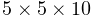and a cell resolution of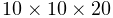, centered at the origin and whose vertex values are set to their distance from the origin, in order to create a simple distance field:

// create a 5 x 5 x 10 grid with resolution 10 x 10 x 20 centered on the
// origin
ScalarGrid grid = new ScalarGrid (
new Vector3d (5, 5, 10), new Vector3i (10, 10, 20));
// use this to create a scalar grid field where the value at each vertex
// is the distance from the origin
ScalarGridField field = new ScalarGridField (grid);
// iterate through all the vertices and set the value for each to its
// distance from the origin, as given by the norm of it’s position
for (int vi=0; vi<grid.numVertices(); vi++) {
Vector3d vpos = grid.getVertexCoords (vi);
grid.setVertexValue (vi, vpos.norm());
}
// add to a mech model:

As shown in the example and as mentioned earlier, grid field are generally added to the fields list of a MechModel.

## 6.10.2 FEM fields

A FEM field specifies scalar or vector values for the features of an FEM mesh. These can be either the nodes (nodal fields), elements (element fields), or element integration points (sub-element fields). As such, FEM fields provide a way to augment the mesh to store application-specific quantities, and are typically used to bind properties of FEM materials that need to vary over the domain (Section 6.10.5).

To evaluate getValue(p) at an arbitrary point, the field finds the FEM element containing(or nearest to, ifis outside the FEM mesh), and either interpolates the value from the surrounding nodes (nodal fields), uses the element value directly (element fields), or interpolates from the integration point values (sub-element fields).

When finding a FEM field value at a point, getValue(p) is evaluated with respect to the FEM model’s current spatial position, as opposed to its rest position.

FEM fields maintain a default value which describes the value at features for which values are not explicitly set. If unspecified, the default value itself is zero.

In the remainder of this section, it is assumed that vector fields are constructed using fixed-size vectors or matrices, such as Vector3d or Matrix3d. However, it is also possible to construct fields using VectorNd or MatrixNd, with the aid of special wrapper classes, as described in Section 6.10.4. That section also details a convenience wrapper class for Vector3d.

The three field types are now described in detail.

### 6.10.2.1 Nodal fields

Implemented by ScalarNodalField, VectorNodalField<T>, or subclasses of the latter, nodal fields specify their values at the nodes of an FEM mesh. They can be created with constructors such as

// scalar fields:
ScalarNodalField (FemModel3d fem)
ScalarNodalField (FemModel3d fem, double defaultValue)
ScalarNodalField (String name, FemModel3d fem, double defaultValue)
// vector fields (with vector type parameterized by T):
VectorNodalField (Class<T> type, FemModel3d fem)
VectorNodalField (Class<T> type, (FemModel3d fem, T defaultValue)
VectorNodalField (String name, Class<T> type, FemModel3d fem, T defaultValue)

where fem is the associated FEM model, defaultValue is the default value, and name is a component name. For vector fields, the maspack.matrix.VectorObject type is parameterized by T, and type gives its actual class type (e.g., Vector3d.class).

Once the grid has been created, values can be queried and set at the nodes using methods such as

// scalar fields:
void setValue (FemNode3d node, double value) // set value for node
double getValue (FemNode3d node)             // get value for node
double getValue (int nodeNum)                // get value using node number
// vector fields:
void setValue (FemNode3d node, T value)      // set value for node
T getValue (FemNode3d node)                  // get value for node
T getValue (int nodeNum)                     // get value using node number
// all fields:
boolean isValueSet (FemNode3d node)          // query if value set for node
void clearValue (FemNode3d node)             // unset value for node
void clearAllValues()                        // unset values for all nodes

Here nodeNum refers to an FEM node’s number (which can be obtained using node.getNumber()). Numbers are used instead of indices to identity FEM nodes and elements because they are guaranteed to be persistent in case of mesh editing, and will remain unchanged as long as the node or element is not removed from the FEM model. Note that values don’t need to be set at all nodes, and set values can be unset using the clear methods. For nodes with no value set, the getValue() methods will return the default value.

As a simple example, the following code fragment constructs a ScalarNodalField for an FEM model fem that describes stiffness values at every node of an FEM:

// instantiate the field and set values for each node:
ScalarNodalField field = new ScalarNodalField ("stiffness", fem);
for (FemNode3d n : fem.getNodes()) {
double stiffness = ... // compute stiffness value for the node
field.setValue (n, stiffness);
}

As noted earlier, FEM fields should be stored in the fields list of the associated FEM model.

Another example shows the creation of a field of 3D direction vectors:

VectorNodalField<Vector3d> field =
new VectorNodalField<Vector3d> ("directions", Vector3d.class, fem);
Vector3d dir = new Vector3d();
for (FemNode3d node : fem.getNodes()) {
... // compute direction and store in dir
field.setValue (node, dir);
}

When creating a vector field, the constructor needs the class type of the field’s VectorObject. However, for the special case of Vector3d, one may also use the convenience wrapper class Vector3dNodalField, described in Section 6.10.4.

### 6.10.2.2 Element fields

Implemented by ScalarElementField, VectorElementField<T>, or subclasses of the latter, element fields specify their values at the elements of an FEM mesh, and these values are assumed to be constant within the element. To evaluate getValue(p), the field finds the containing (or nearest) element for, and then returns the value for that element.

Because values are assume to be constant within each element, element fields are inherently discontinuous across element boundaries.

The constructors for element fields are analogous to those for nodal fields:

// scalar fields:
ScalarElementField (FemModel3d fem)
ScalarElementField (FemModel3d fem, double defaultValue)
ScalarElementField (String name, FemModel3d fem, double defaultValue)
// vector fields (with vector type parameterized by T):
VectorElementField (Class<T> type, FemModel3d fem)
VectorElementField (Class<T> type, (FemModel3d fem, T defaultValue)
VectorElementField (String name, Class<T> type, FemModel3d fem, T defaultValue)

and the methods for setting values at elements are similar as well:

// scalar fields:
void setValue (FemElement3dBase elem, double value) // set value for element
double getValue (FemElement3dBase elem)      // get value for element
double getElementValue (int elemNum)         // get value for volume element
double getShellElementValue (int elemNum)    // get value for shell element
// vector fields:
void setValue (FemElement3dBase elem, T value) // set value for element
T getValue (FemElement3dBase elem)           // get value for element
T getElementValue (int elemNum)              // get value for volume element
T getShellElementValue (int elemNum)         // get value for shell element
// all fields:
boolean isValueSet (FemElement3dBase elem)   // query if value set for element
void clearValue (FemElement3dBase elem)      // unset value for element
void clearAllValues()                        // unset values for all elements

The elements associated with an element field can be either volume or shell elements, which are stored in the FEM component lists elements and shellElements, respectively. Since volume and shell elements may share element numbers, the separate methods getElementValue() and getShellElementValue() are used to access values by element number.

The following code fragment constructs a VectorElementField based on Vector2d that stores a 2D coordinate value at all regular and shell elements:

VectorElementField<Vector2d> efield =
new VectorElementField<Vector2d> ("coordinates", Vector2d.class, fem);
Vector2d coords = new Vector2d();
for (FemElement3d e : fem.getElements()) {
... // compute coordinate values and store in coords
efield.setValue (e, coords);
}
for (ShellElement3d e : fem.getShellElements()) {
... // compute coordinate values and store in coords
efield.setValue (e, coords);
}

### 6.10.2.3 Sub-element fields

Implemented by ScalarSubElemField, VectorSubElemField<T>, or subclasses of the latter, sub-element fields specify their values at the integration points within each element of an FEM mesh. These fields are used when we need precisely computed information at each of the element’s integration points, and we can’t assume that nodal interpolation will give an accurate enough approximation.

To evaluate getValue(p), the field finds the containing (or nearest) element for, extrapolating the intergration point values back to the nodes, and then using nodal interpolation.

Constructors are similar to those for element fields:

// scalar fields:
ScalarSubElemField (FemModel3d fem)
ScalarSubElemField (FemModel3d fem, double defaultValue)
ScalarSubElemField (String name, FemModel3d fem, double defaultValue)
// vector fields:
VectorSubElemField (Class<T> type, FemModel3d fem)
VectorSubElemField (Class<T> type, (FemModel3d fem, T defaultValue)
VectorSubElemField (String name, Class<T> type, FemModel3d fem, T defaultValue)

Value accessors are also similar, except that an additional argument k is required to specify the index of the integration point:

// scalar fields:
void setValue (FemElement3dBase e, int k, double value) // set value for point
double getValue (int elemNum, int k)               // get value for point
double getElementValue (FemElement3dBase e, int k) // get value for volume point
double getShellElementValue (int elemNum, int k)   // get value for shell point
// vector fields:
void setValue (FemElement3dBase e, T value, int k) // set value for point
T getValue (FemElement3dBase e, int k)             // get value for point
T getElementValue (int elemNum, int k)             // get value for volume point
T getShellElementValue (int elemNum, int k)        // get value for shell point
// all fields:
boolean isValueSet (FemElement3dBase e, int k)     // query if value set for point
void clearValue (FemElement3dBase e, int k)        // unset value for point
void clearAllValues()                              // unset values for all points

The integration point index k should be in the range 0 to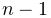, where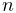is the total number of integration points for the element in question and can be queried by the method numAllIntegrationPoints().

The total number of integration pointsincludes both the regular integration point as well as the warping point, which is located at the element center, is indexed by, and is used for corotated linear materials. If a SubElemField is being using to supply mesh-varying values to one of the linear material parameters (Section 6.10.5), then it is important to supply values at the warping point.

The example below shows the construction of a ScalarSubElemField:

ScalarSubElemField field = new ScalarSubElemField ("modulus", fem);
for (FemElement3d e : fem.getElements()) {
IntegrationPoint3d[] ipnts = e.getAllIntegrationPoints();
for (int k=0; k<ipnts.length; k++) {
double value = ... // compute value at integration point k
field.setValue (e, k, value);
}
}

First, the field is instantiated with the name "modulus". The code then iterates first through the FEM elements, and then through each element’s integration points, computing values appropriate to each one. A list of each element’s integration points is returned by e.getAllIntegrationPoints(). Having access to the actual integration points is useful in case information about them is needed to compute the field values. In particular, if it is necessary to obtain an integration point’s rest or current (spatial) position, these can be queried as follows:

IntegrationPoint3d ipnt;
Point3d pos = new Point3d();
FemElement3d elem;
...
// compute spatial position:
ipnt.computePosition (pos, elem.getNodes());
// compute rest position:
ipnt.computeRestPosition (pos, elem.getNodes());

The regular integration points, excluding the warping point, can be queried using getIntegrationPoints() and numIntergrationPoints() instead of getAllIntegrationPoints() and numAllIntegrationPoints().

## 6.10.3 Mesh fields

A mesh field specifies scalar or vector values for the features of an FEM mesh. These can be either the vertices (vertex fields) or faces (face fields). Mesh fields have been introduced in particular to allow properties of contact force behaviors (Section 7.7.2), such as LinearElasticContact, to vary over the contact mesh. Mesh fields can currently be defined only for triangular polyhedral meshes.

To evaluate getValue(p) at an arbitrary point, the field finds the mesh face nearest to, and then either interpolates the value from the surrounding vertices (vertex fields) or uses the face value directly (face fields).

Mesh fields make use of the classes PolygonalMesh, Vertex3d, and Face, defined in the package maspack.geometry. They maintain a default value which describes the value at features for which values are not explicitly set. If unspecified, the default value itself is zero.

In the remainder of this section, it is assumed that vector fields are constructed using fixed-size vectors or matrices, such as Vector3d or Matrix3d. However, it is also possible to construct fields using VectorNd or MatrixNd, with the aid of special wrapper classes, as described in Section 6.10.4. That section also details a convenience wrapper class for Vector3d.

The two field types are now described in detail.

### 6.10.3.1 Vertex fields

Implemented by ScalarVertexField, VectorVertexField<T>, or subclasses of the latter, vertex fields specify their values at the vertices of a mesh. They can be created with constructors such as

// scalar fields:
ScalarVertexField (MeshComponent mcomp)
ScalarVertexField (MeshComponent mcomp, double defaultValue)
ScalarVertexField (String name, MeshComponent mcomp, double defaultValue)
// vector fields (with vector type parameterized by T):
VectorVertexField (Class<T> type, MeshComponent mcomp)
VectorVertexField (Class<T> type, (MeshComponent mcomp, T defaultValue)
VectorVertexField (String name, Class<T> type, MeshComponent mcomp, T defaultValue)

where mcomp is a mesh component containing the mesh, defaultValue is the default value, and name is a component name. For vector fields, the maspack.matrix.VectorObject type is parameterized by T, and type gives its actual class type (e.g., Vector3d.class).

Once the field has been created, values can be queried and set at the vertices using methods such as

// scalar fields:
void setValue (Vertex3d vtx, double value)   // set value for vertex
double getValue (Vertex3d vtx)               // get value for vertex
double getValue (int vidx)                   // get value for vertex number
// vector fields:
void setValue (Vertex3d vtx, T value)        // set value for vertex
T getValue (Vertex3d vtx)                    // get value for vertex
T getValue (int vidx)                        // get value for vertex number
// all fields:
boolean isValueSet (Vertex3d vtx)            // query if value set for vertex
void clearValue (Vertex3d vtx)               // unset value for vertex
void clearAllValues()                        // unset values for all vertices

where vidx is the vertex’s index. Note that values don’t need to be set at all vertices, and set values can be unset using the clear methods. For vertices with no value set, getValue() will return the default value.

As a simple example, the following code fragment constructs a ScalarVertexField for a mesh contained in the MeshComponent mcomp that describes contact stiffness values at every vertex:

MechModel mech; // containing mech model
...
// instantiate the field and set values for each node:
ScalarVertexField field = new ScalarVertexField ("stiffness", mcomp);
// extract the mesh from the component
PolygonalMesh mesh = (PolygonalMesh)mcomp.getMesh();
for (Vertex3d vtx : mesh.getVertices()) {
double stiffness = ... // compute stiffness value for the vertex
field.setValue (vtx, stiffness);
}

As noted earlier, mesh fields are usually stored in the fields list of a MechModel.

Another example shows the creation of a field of 3D direction vectors:

VectorVertexField<Vector3d> field =
new VectorVertexField<Vector3d> ("directions", Vector3d.class, mcomp);
Vector3d dir = new Vector3d();
for (Vertex3d vtx : mesh.getVertices()) {
... // compute direction and store in dir
field.setValue (vtx, dir);
}

When creating a vector field, the constructor needs the class type of the field’s VectorObject. However, for the special case of Vector3d, one may also use the convenience wrapper class Vector3dVertexField, described in Section 6.10.4.

### 6.10.3.2 Face fields

Implemented by ScalarFaceField, VectorFaceField<T>, or subclasses of the latter, face fields specify their values at the faces of a polygonal mesh, and these values are assumed to be constant within the face. To evaluate getValue(p), the field finds the containing (or nearest) face for, and then returns the value for that face.

Because values are assume to be constant within each face, face fields are inherently discontinuous across face boundaries.

The constructors for face fields are analogous to those for vertex fields:

// scalar fields:
ScalarFaceField (MeshComponent mcomp)
ScalarFaceField (MeshComponent mcomp, double defaultValue)
ScalarFaceField (String name, MeshComponent mcomp, double defaultValue)
// vector fields (with vector type parameterized by T):
VectorFaceField (Class<T> type, MeshComponent mcomp)
VectorFaceField (Class<T> type, (MeshComponent mcomp, T defaultValue)
VectorFaceField (String name, Class<T> type, MeshComponent mcomp, T defaultValue)

and the methods for setting values at faces are similar as well, where fidx refers to the face index:

// scalar fields:
void setValue (Face face, double value)      // set value for face
double getValue (Face face)                  // get value for face
double getValue (int fidx)                   // get value using face index
// vector fields:
void setValue (Face face, T value)           // set value for face
T getValue (Face face)                       // get value for face
T getValue (int fidx)                        // get value using face index
// all fields:
boolean isValueSet (Face face)               // query if value set for face
void clearValue (Face face)                  // unset value for face
void clearAllValues()                        // unset values for all faces

The following code fragment constructs a VectorFaceField based on Vector2d that stores a 2D coordinate value at all faces of a mesh:

VectorFaceField<Vector2d> field =
new VectorFaceField<Vector2d> ("coordinates", Vector2d.class, mcomp);
Vector2d coords = new Vector2d();
// extract the mesh from the component
PolygonalMesh mesh = (PolygonalMesh)mcomp.getMesh();
for (Face face : mesh.getFaces()) {
... // compute coordinate values and store in coords
field.setValue (face, coords);
}

## 6.10.4 Fields for VectorNd, MatrixNd and Vector3d

The vector fields described above can be implemented for most fixed-size vectors and matrices defined in the package maspack.matrix (e.g., Vector2d, Vector3d, Matrix3d). However, if vectors or matrices with different size are needed, it is also possible to create vector fields using VectorNd and MatrixNd, provided that the sizing remain constant within any given field. This is acheived using special wrapper classes that contain the sizing information, which is supplied in the constructors. For convenience, wrapper classes are also provided for vector fields that use Vector3d, since that vector size is quite common.

For vector FEM and mesh fields, the complete set of wrapper classes is listed below:

Class Vector FEM fields: base class vector type T VectorNodalField Vector3d VectorNodalField VectorNd VectorNodalField MatrixNd VectorElementField Vector3d VectorElementField VectorNd VectorElementField MatrixNd VectorSubElemField Vector3d VectorSubElemField VectorNd VectorSubElemField MatrixNd VectorVertexField Vector3d VectorVertexField VectorNd VectorVertexField MatrixNd VectorFaceField Vector3d VectorFaceField VectorNd VectorFaceField MatrixNd

These all behave identically to their base classes, except that their constructors omit the type argument (since this is built into the class definition) and, for VectorNd and MatrixNd, supply information about the vector or matrix sizes. For example, constructors for the FEM nodal fields include

Vector3dNodalField (FemModel3d fem)
Vector3dNodalField (FemModel3d fem, Vector3d defaultValue)
Vector3dNodalField (String name, FemModel3d fem, Vector3d defaultValue)
VectorNdNodalField (int vecSize, FemModel3d fem)
VectorNdNodalField (int vecSize, FemModel3d fem, VectorNd defaultValue)
VectorNdNodalField (
int vecSize, String name, FemModel3d fem, VectorNd defaultValue)
MatrixNdNodalField (int rowSize, int colSize, FemModel3d fem)
MatrixNdNodalField (
int rowSize, int colSize, FemModel3d fem, MatrixNd defaultValue)
MatrixNdNodalField (
int rowSize, int colSize, String name, FemModel3d fem, MatrixNd defaultValue)

where vecSize, rowSize and colSize give the sizes of the VectorNd or MatrixNd objects within the field. Constructors for other field types are equivalent and are described in their API documentation.

For grid fields, one can use either VectorNdGrid or MatrixNdGrid, both defined in maspack.geometry, as the primary grid used to construct the field. Constructors for these include

VectorNdGrid (int VecSize, Vector3d widths, Vector3i res)
MatrixNdGrid (
int rowSizem int colSize, Vector3d widths, Vector3i res, RigidTransform3d TCL)

## 6.10.5 Binding material properties

A principal purpose of field components is to enable certain FEM material properties to vary spatially over the FEM geometry. Many material properties can be bound to a field, so that when they are queried internally by the solver at the integration points for each FEM element, the field value at that integration point is used instead of the regular property value. Likewise, some properties of contact force behaviors, such as the YoungsModulus and thickness properties of LinearElasticContact (Section 7.7.3) can be bound to a field that varies over the contact mesh geometry.

To bind a property to a field, it is necessary that

1. 1.

The type of the field matches the value of the property;

2. 2.

The property is itself bindable.

If the property has a double value, then it can be bound to any ScalarFieldComponent. Otherwise, if the property has a value T, where T is an instance of maspack.matrix.VectorObject, then it can be bound to a vector field.

Bindable properties export two methods with the signature

setXXXField (F field)
F getXXXField ()

where XXX is the property name and F is an appropriate field type.

As long as a property XXX is bound to a field, its regular value will still appear (and can be set) through widgets in control or property panels, or via its getXXX() and setXXX() accessors. However, the regular value won’t be used internally by the FEM simulation.

For example, consider the YoungsModulus property, which is present in several FEM materials, including LinearMaterial and NeoHookeanMaterial. This has a double value, and is bindable, and so can be bound to a ScalarFieldComponent as follows:

FemModel3d fem;
ScalarNodalField field;
NeoHookeanMaterial mat;
... other initialization ...
mat.setYoungsModulusField (field);  // bind to the field
fem.setMaterial (mat);   // set the material in the FEM model

It is important to perform field bindings on materials before they are set in an FEM model (or one of its subcomponents, such as MuscleBundles). That’s because the setMaterial() method copies the input material, and so any settings made on it afterwards won’t be seen by the FEM:

   // works: field will be seen by the copied version of ’mat’
mat.setYoungsModulusField (field);
fem.setMaterial (mat);

// does NOT work: field not seen by the copied version of ’mat’
fem.setMaterial (mat);
mat.setYoungsModulusField (field);


To unbind YoungsModulus, one can call

mat.setYoungsModulusField (null);

Usually, FEM fields are used to bind properties in FEM materials while mesh fields are used for contact properties, but other fields can be used, particularly grid fields. To understand this a bit better, we discuss briefly some of the internals of how binding works. When evaluating stresses, FEM materials have access to a FemFieldPoint object that provides information about the integation point, including its element, nodal weights, and integration point index. This can be used to rapidly evaluate values within nodal, element and sub-element FEM fields. Likewise, when evaluating contact forces, contact materials like LinearElasticContact have access to a MeshFieldPoint object that describes the contact point position as a weighted sum of mesh vertex positions, which can then be used to rapidly evaluate evaluate values within vertex or face mesh fields. However, all fields, regardless of type, implement methods for determining values at both FemFieldPoints and MeshFieldPoints:

T getValue (FemFieldPoint fp)
T getValue (MeshFieldPoint mp)

If necessay, these methods simply fall back on the getValue(Point3d) method, which is defined for all fields and works relatively fast for grid fields.

There are some additional details to consider when binding FEM material properties:

1. 1.

When binding to a grid field, one has the choice of whether to use the grid to represent values with respect to the FEM’s rest position or spatial position. This can be controlled by setting the useFemRestPositions property of the grid field, the default value for which is true.

2. 2.

When binding the bulkModulus property of an incompressible material, it is best to use a nodal field if the FEM model is using nodal-based soft incompressibility (i.e., the model’s softIncompMethod property is set to either NODAL or AUTO; Section 6.7.3). That’s because soft nodal incompressibility require evaluating the bulk modulus property at nodes instead of integration points, which is much easier to do with a nodal-based field.

## 6.10.6 Example: FEM with variable stiffness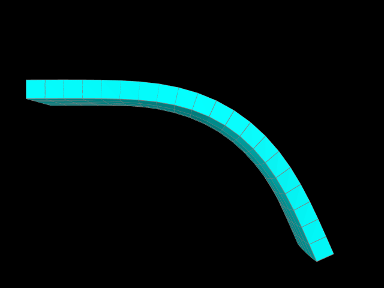Figure 6.15: VariableStiffness model after being run in ArtiSynth.

A simple model demonstrating a stiffness that varies over an FEM mesh is defined in

  artisynth.demos.tutorial.VariableStiffness


It consists of a simple thin hexahedral beam with a linear material for which the Young’s modulus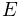is made to vary nonlinearly along the x axis of the rest position according to the formula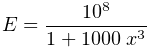(6.10)

The model’s build method is given below:

1    public void build (String[] args) {
2       MechModel mech = new MechModel ("mech");
4
5       // create regular hex grid FEM model
6       FemModel3d fem = FemFactory.createHexGrid (
7          null, 1.0, 0.25, 0.05, 20, 5, 1);
8       fem.transformGeometry (new RigidTransform3d (0.5, 0, 0)); // shift right
9       fem.setDensity (1000.0);
11
12       // fix the left-most nodes
13       double EPS = 1e-8;
14       for (FemNode3d n : fem.getNodes()) {
15          if (n.getPosition().x < EPS) {
16             n.setDynamic (false);
17          }
18       }
19       // create a scalar nodel field to make the stiffness vary
20       // nonlinearly along the rest position x axis
21       ScalarNodalField stiffnessField = new ScalarNodalField(fem, 0);
22       for (FemNode3d n : fem.getNodes()) {
23          double s = 10*(n.getRestPosition().x);
24          double E = 100000000*(1/(1+s*s*s));
25          stiffnessField.setValue (n, E);
26       }
28       // create a linear material, bind its Youngs modulus property to the
29       // field, and set the material in the FEM model
30       LinearMaterial linearMat = new LinearMaterial (100000, 0.49);
31       linearMat.setYoungsModulusField (stiffnessField); //, /*useRestPos=*/true);
32       fem.setMaterial (linearMat);
33
34       // set some render properties for the FEM model
36       RenderProps.setFaceColor (fem, Color.CYAN);
37    }

Lines 6-10 create the hex FEM model and shift it so that the left side is aligned with the origin, while lines 12-17 fix the leftmost nodes. Lines 21-27 create a scalar nodal field for the Young’s modulus, with lines 23-24 computingaccording to (6.10). The field is then bound to a linear material which is then set in the model (lines 30-32).

The example can be run in ArtiSynth by selecting All demos > tutorial > VariableStiffness from the Models menu. When run, the beam will bend under gravity, but mostly on the right side, due to the much higher stiffness on the left (Figure 6.15).

## 6.10.7 Example: specifying FEM muscle directions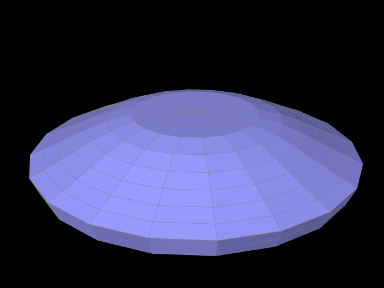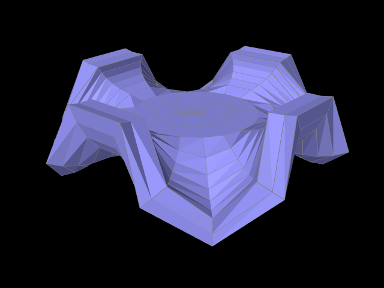Figure 6.16: (Top) RadialMuscle model after being loaded into ArtiSynth and run with its excitation set to 0. (Bottom) RadialMuscle with its excitation set to around .375.

Another example involves using a Vector3d field to specify the muscle activation directions over an FEM model and is defined in

  artisynth.demos.tutorial.RadialMuscle


When muscles are added using MuscleBundles ( Section 6.9), the muscle directions are stored and handled internally by the muscle bundle itself. However, it is possible to add a MuscleMaterial directly to the elements of an FEM model, using a MaterialBundle (Section 6.8), in which case the directions need to be set explicitly using a field.

The model’s build method is given below:

1    public void build (String[] args) {
2       MechModel mech = new MechModel ("mech");
4
5       // create a thin cylindrical FEM model with two layers along z
7       FemMuscleModel fem = new FemMuscleModel ("radialMuscle");
9       fem.setDensity (1000);
11       fem.setMaterial (new NeoHookeanMaterial (200000.0, 0.33));
12       // fix the nodes close to the center
13       for (FemNode3d node : fem.getNodes()) {
14          Point3d pos = node.getPosition();
15          double radialDist = Math.sqrt (pos.x*pos.x + pos.y*pos.y);
17             node.setDynamic (false);
18          }
19       }
20       // compute a direction field, with the directions arranged radially
21       Vector3d dir = new Vector3d();
22       Vector3dElementField dirField = new Vector3dElementField (fem);
23       for (FemElement3d elem : fem.getElements()) {
24          elem.computeCentroid (dir);
25          // set directions only for the upper layer elements
26          if (dir.z > 0) {
27             dir.z = 0; // remove z component from direction
28             dir.normalize();
29             dirField.setValue (elem, dir);
30          }
31       }
33       // add a muscle material, and use it to hold a simple force
34       // muscle whose ’restDir’ property is attached to the field
35       MaterialBundle bun = new MaterialBundle ("bundle",/*all elements=*/true);
37       SimpleForceMuscle muscleMat = new SimpleForceMuscle (500000);
38       muscleMat.setRestDirField (dirField);
39       bun.setMaterial (muscleMat);
40
41       // add a control panel to control the excitation
42       ControlPanel panel = new ControlPanel();
43       panel.addWidget (bun, "material.excitation", 0, 1);
45
46       // set some rendering properties
Lines 5-19 create a thin cylindrical FEM model, centered on the origin, with radius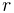and height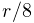, consisting of hexes with wedges at the center, with two layers of elements along theaxis (which is parallel to the cylinder axis). Its base material is set to a neo-hookean material. To keep the model from falling under gravity, all nodes whose distance to theaxis is less than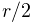are fixed.
Next, a Vector3d field is created to specify the directions, on a per-element basis, for the muscle material which will be added subsequently (lines 21-32). While we could create an instance of VectorElementField<Vector3d>, we use Vector3dElementField, since this is available and provides additional functionality (such as the ability to render the directions). Directions are set to lie outward in a radial direction perpendicular to theaxis, and since the model is centered on the origin, they can be computed easily by first computing the element centroids, removing thecomponent, and then normalizing. In order to give the muscle action an upward bias, we only set directions for elements in the upper layer. Direction values for elements in the lower layer will then automatically have a default value of 0, which will cause the muscle material to not apply any stress.